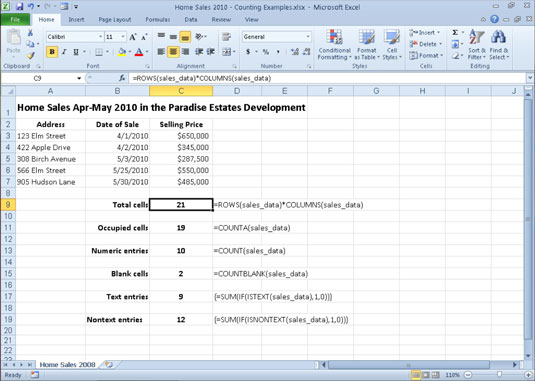##### Excel 2010 All-in-One For DummiesExcel 2010 offers an assortment of counting functions — including COUNT, COUNTA, COUNTBLANK — in the Statistical category that enable you to count the number of cells that contain numeric values, that are nonblank (and thus contain entries of any kind), or whose values meet the criteria that you specify. You can find these functions on the Statistical continuation menu accessed from the More Functions button's drop-down menu on the Formulas tab.

The following figure illustrates the different types of counting formulas that you can build to return such basic statistics as the total number of cells in a particular range, the number of occupied cells in that range, and the number of numeric and text entries in the occupied range. In this worksheet, the cell range A1:C8 has been assigned the name sales_data.A home sales worksheet that uses various counting functions.

The most basic formula is the one that returns the total number of cells in the sales_data range. To build this formula in cell C9, use the ROWS and COLUMNS information functions to return the number of rows and columns in the range, and then create the following formula that multiplies these two values together:

`=ROWS(sales_data)*COLUMNS(sales_data)`

This formula, of course, returns 21 to cell C9. The next formula calculates the number of these 21 cells that contain data entries (of whatever type) using the COUNTA function. This function counts the number of cells that are not empty in the ranges that you specify. The COUNTA function uses the following syntax:

`=COUNTA(value1,[<em>value2</em>],[...])`

The value arguments (all of which are optional except for value1) include up to 30 different values or cell ranges that you want counted. In the example shown in the figure, cell C11 contains the following COUNTA function:

`=COUNTA(sales_data)`

The next formula in the sample worksheet calculates the number of numeric entries in the cell range sales_data. To do this, you use the COUNT function. The COUNT function takes the same arguments as COUNTA, the only difference being that COUNT counts a value or cell specified in its value arguments only if it contains a numeric entry.

Cell C13 contains the following formula for calculating the number of numeric entries in the Home Sales table range called sales_data:

`=COUNT(sales_data)`

Note that in calculating this result, Excel counts the five date entries (with the date of each sale) in the cell range B3:B7 as well as the five numeric data entries (with the selling prices of each home) in the cell range C3:C7.

The next formula in the sample worksheet uses the COUNTBLANK function to calculate the number of blank cells in the sales_data range. This example uses the following COUNTBLANK function in cell C15:

`=COUNTBLANK(sales_data)`